## Introduction

The search for more efficient optimization algorithms is an important endeavor with prevalence on many disciplines ranging from the social sciences to the physical and natural sciences. Belonging to the latter, the protein folding problem1,2,3,4,5,6,7 consists of finding the lowest free-energy configuration or, equivalently, the native structure of a protein given its amino-acid sequence. Knowing how proteins fold elucidate their three-dimensional structure-function relationship which is crucial to the understanding of enzymes and for the treatment of misfolded-protein diseases such as Alzheimer's, Huntington's and Parkinson's disease. Due to the high computational cost of modeling proteins in atomistic detail8,9, coarse-grained descriptions of the protein folding problem, such as those found in lattice models, provide valuable insight about the folding mechanisms2,4,5,6,10.

Harnessing quantum-mechanical effects to speed up the solving of classical optimization problems is at the heart of quantum annealing algorithms (QA)11,12,13,14,15. There is theoretical11,12,16,17,18 and experimental19 evidence of the advantage of solving classical optimization problems using QA11,12,13,14 over its classical analogue (simulated annealing20). In QA, quantum mechanical tunneling allows for more efficient exploration of difficult potential energy landscapes such as that of classical spin-glass problems. In our implementation of lattice folding, quantum fluctuations (tunneling) occurs between states representing different model protein conformations or folds.

The theoretical challenge is to efficiently map the hard computational problem of interest (e.g., lattice folding) to a classical spin-glass Hamiltonian: such mapping requiring a polynomial number of quantum bits (qubits) with the size of the problem (protein length) is described elsewhere21. Here we present a new mapping which, due to its exponential scaling with problem size, is not intended for large instances. The proposed mapping employs very few qubits for small problem instances, making it ideal for this first experimental demonstration and implementation on current quantum devices22. A combination of the existing polynomial mapping21 and more advanced quantum devices would allow for the simulation of much larger instances of lattice folding and other related optimization problems.

Solving arbitrary problem instances requires a programmable quantum device to implement the corresponding classical Hamiltonian. We employ quantum annealing on the programmable device to obtain low-energy conformations of the protein model. We emphasize that nothing quantum mechanical is implied about the protein or its folding process; rather quantum fluctuations are a tool we use to solve the optimization problem.

The QA protocol performed here is also known as adiabatic quantum computation (AQC)17,23. Of all the quantum-computational models, AQC is perhaps the most naturally suited for studying and solving optimization problems17,24. For the experiments presented here, the small finite temperature of the superconducting device is enough to make the process less coherent than the original formulation of AQC, where the theoretical limit of zero temperature and quasi-adiabaticity are usually assumed17,23. As we show in the discussion, numerical simulations including these unavoidable environmental effects accurately reproduce our experimental results.

Experimental implementations of QA or AQC are limited either by the number of qubits available in state-of-the-art quantum devices or by the programmability required to fulfill the problem specification. For example, the first realization of AQC was performed on a three-qubit NMR quantum device25 and newer NMR implementations involve four qubit experiments26. Other experimental realizations of spin systems have been based on measuring bulk magnetization properties of the systems in which there is no control over the individual spins and the couplings among them19,27,28. Quantum architectures using superconducting qubits29,30,31,32,33,34,35,36 offer promising device scalability while maintaining the ability to control individual qubits and the strength of their interaction couplings. During the preparation of this manuscript, an 84-qubit experimental determination of Ramsey numbers with quantum annealing was performed37, underscoring the programmable capabilities of the device for problems with over 80 qubits. In this letter, we present a quantum annealing experimental implementation of lattice protein models with general (Miyazawa-Jernigan38) interactions among the amino acids. Even though the cases presented here still can be solved on a classical computer by exact enumeration (the six-amino-acid problem has only 40 possible configurations), it is remarkable that the device anneals to the ground state of a search space of 281 possible computational outcomes. This study provides a proof-of-principle that optimization of biophysical problems such as protein folding can be studied using quantum mechanical devices.

## Results

The quantum hardware employed consists of 16 units of a recently characterized eightqubit unit cell22,39. Post-fabrication characterization determined that only 115 qubits out of the 128 qubit array can be reliably used for computation (see Fig. 1). The array of coupled superconducting flux qubits is, effectively, an artificial Ising spin system with programmable spin-spin couplings and transverse magnetic fields. It is designed to solve instances of the following (NP-hard40) classical optimization problem: Given a set of local longitudinal fields {hi} and an interaction matrix {Jij}, find the assignment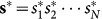, that minimizes the objective function E(s), where,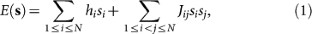|hi| ≤ 1, |Jij| ≤ 1 and si {+1, -1}.

Finding the optimal s* is equivalent to finding the ground state of the corresponding Ising classical Hamiltonian,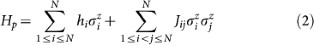where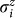are Pauli matrices acting on the ith spin.

Experimentally, the time-dependent quantum Hamiltonian implemented in the superconductingqubit array is given by,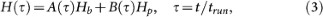with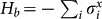responsible for quantum tunneling among the localized classical states, which correspond to the eigenstates of Hp (the computational basis). The time-dependent functions A(τ) and B(τ) are such that A(0) B(0) and A(1) B(1); in Fig. 2(b), we plot these functions as implemented in the experiment. trun denotes the time elapsed between the preparation of the initial state and the measurement.

QA exploits the adiabatic theorem of quantum mechanics, which states that a quantum system initialized in the ground state of a time-dependent Hamiltonian remains in the instantaneous ground state, as long as it is driven sufficiently slowly. Since the ground state of Hp encodes the solution to the optimization problem, the idea behind QA is to adiabatically prepare this ground state by initializing the quantum system in the easy-to-prepare ground state of Hb, which corresponds to a superposition of all 2N states of the computational basis. The system is driven slowly to the problem Hamiltonian, H(τ = 1) ≈ Hp. Deviations from the ground-state are expected due to deviations from adiabaticity, as well as thermal noise and imperfections in the implementation of the Hamiltonian.

The first challenge of the experimental implementation is to map the computational problem of interest into the binary quadratic expression (Eq. 2), which we outline next. In lattice folding, the sequence of amino acids defining the protein is viewed as a sequence of beads (amino acids) connected by strings (peptide bonds). This bead chain occupies points on a two- or three-dimensional lattice. A valid configuration is a self-avoiding walk on the lattice and its energy is calculated from the sum of interaction energies between nearest non-bonded neighbors on the lattice. By the thermodynamic hypothesis of protein folding41, the global minimum of the free-energy function is conjectured to be the native functional conformation of the protein.

Finding low-energy three-dimensional structures is an intractable problem42,43,44. The hydrophobic-polar (HP) model is one of the simplest possible models for lattice folding45. In this model, the amino acids are classified into two groups, hydrophobic (H) and polar (P). Even in this simplest model, exhaustive search of all possible global minima is limited to sequences in the tens of amino acids46. To describe real protein energy landscapes a more elaborate description needs to be considered, such as the Mijazawa-Jernigan (MJ) model38 which assigns the interaction energies for pairwise interactions among all twenty amino acids. The formulation we used is general enough to take into account arbitrary interaction matrices for lattice models in two and three dimensions. In particular, we solved a MJ model in 2D, the six-amino-acid sequence of Proline-Serine-Valine-Lysine-Methionine-Alanine (PSVKMA in the one-letter amino-acid sequence notation). We solved the problem under two different experimental schemes (see Schemes 2 and 3 in Fig. 3), each requiring a different number of resources. Solving the problem in one proposed experimental realization (Scheme 1) requires more resources than the number of qubits available (115 qubits) in the device. Scheme 2 and 3 are examples of the divide-and-conquer strategy, in which one partitions the problem in smaller instances and combines the independent set of results, thereby obtaining the same solution for the intractable problem. In the Supplementary Information section, we complement these four MJ related experiments with two small tetrapeptide instances (effectively HP model instances) for a total of six different problem Hamiltonians. We used the largest of these two instances (an 8 qubit experiment) for direct theoretical simulation of the annealing dynamics of the device. The results from our experiment and the theoretical model, which does not use any adjustable parameters (all are extracted experimentally from the device), are in excellent agreement (see Supplementary Fig. S2 online).

To represent each of the possible N-amino-acid configurations (folds) in the lattice, we encode the direction of each successive bond between amino acids; thus, for every N-bead sequence we need to specify N - 1 turns corresponding to the number of bonds. For the case of a two dimensional lattice, a bond can take any of four possible directions; therefore, two bits per bond are required to uniquely determine a direction. More specifically, if a bond points upwards, we write “11”. If it points downwards, leftwards or rightwards, we write “00”, “10”, or “01” respectively. Fixing the direction of the first bond reduces the description of any N-bead fold to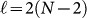binary variables, without loss of generality. As shown in Fig. 2(a), in the absence of external constraints other than those imposed by the primary amino-acid sequence (see Supplementary Information for an example with external constraints), we can fix the third binary variable to “0”, forcing the third amino acid to go either straight or downward and reducing the number of needed variables to. This constraint reduces the solution space by removing conformations which are degenerate due to rotational symmetry. Thus, a particular fold is uniquely defined by,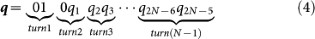An example of this encoding for a six-amino-acid sequence is represented in Fig. 2(a).

Using this mapping to translate between the amino-acid chain in the lattice and the 2(N - 1) string of bits, we constructed the energy function E(q) in which q denotes the remaining 2N-5 binary variables. Additionally, we penalized folds which exhibit two amino acids on top of each other, to favor self-avoiding walk configurations. The energy penalty chosen for each problem was sufficient to push the energy of invalid folds outside of the energy range of valid configurations (those with E ≤ 0). Finally, we took into account the interaction energy among the different amino acids. A detailed construction of our energy function for the general case of N amino acids with arbitrary interactions is given elsewhere.

The experiment consists of the following steps: a) construction of the energy function to be minimized in terms of the turn encoding; b) reduction of the energy expression to a two-body Hamiltonian; and finally, c) embedding in the device. These last two steps need additional resources as explained below. We will focus on the simplest example (Experiment 3, Fig. 3) to show the procedure in detail. The embeddings for the other five experiments are provided in the Supplementary material. The energy function for Experiment 3, containing the contributions due to on-site penalties for overlapping amino acids and pairwise interactions between amino acids is,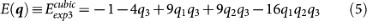where q10 (q2q3) encodes the orientation of the fourth (fifth) bond (see Fig. 3). From Eq. 5 one can verify by substitution that the eight possible three-bit-variable assignments provide the desired energy landscape: the six conformations with E ≤ 0 shown in blue in Fig. 3.

Eq. 5 describes the energy landscape of configurations but it is not quite ready for the device. Experimentally, we can specify up to two-body spin interactions,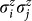and therefore, we need to convert this cubic energy function (Eq. 5) into a quadratic form resembling Eq. 1 (see Supplementary Information for details). The resulting expression iswhere the original binary variables and spin operators are related by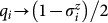. Experimental measurements ofyield si = +1 (si = −1) corresponding to qi = 0 (qi = 1). Since qi = (1 − si)/2, measurement of s1, s2 and s3 allows us to reconstruct the bit string q10q2q3 which encodes the desired fold.

One ancilla variable was added during the transformation of the three-variable cubic Hamiltonian into this quadratic four-variable expression. The meaning of the original variables s1, s2 and s3 remains the same, allowing for the reconstruction of the folds. The energy of this four-variable expression will not change as long as the measurements of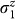through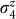result in values for q1q2q3q4 satisfying q4 = q2q3. This transformation ensures an energy penalty whenever this condition is violated.

The architecture of the chip lacks sufficient connectivity between the superconducting rings for a one-to-one assignment of variables to qubits (see Fig. 4). To satisfy the connectivity requirements of the four-variable energy function, the couplings of one of the most connected variables, q4, were fulfilled by duplicating this variable inside the device such that q4q4 and q4′. In the form of Eq. 2 the final expression representing the energy function of Experiment 3 is given by,This expression satisfies all requirements for the problem Hamiltonian (Eq. 3), the completion of which allows for the measurement of the energetic minimum conformation of this small peptide instance. The embedding of Eq. 7 into the hardware is shown in Fig. 4, where we label the five qubits used, q1, q2, q3, q4 and q4′. Since we want the two qubits representing q4 to end up with the same value, we apply the maximum ferromagnetic coupling (J = −1) between them, which adds a penalty whenever this equality is violated (last term in Eq. 7). These maximum couplings are indicated in Fig. 4 by heavy lines. The thinner lines show the remaining couplings used to realize the quadratic terms in Eq. 7, color coded according to the sign of the interaction and its thickness representing their strength. Note that every quadratic term in Eq. 7 has a corresponding coupler. Hereafter, we will denote the outcome of the five-qubit measurements as qexp3 = 010010q10q2q3|q4q4′, with qi = 0 (qi = 1) whenever si = 1 (si = −1). Notice that only the bits preceding the divider character | contain physical information. These are the ones shown under each of the protein fold drawings associated with Experiment 3 (see Fig. 3).